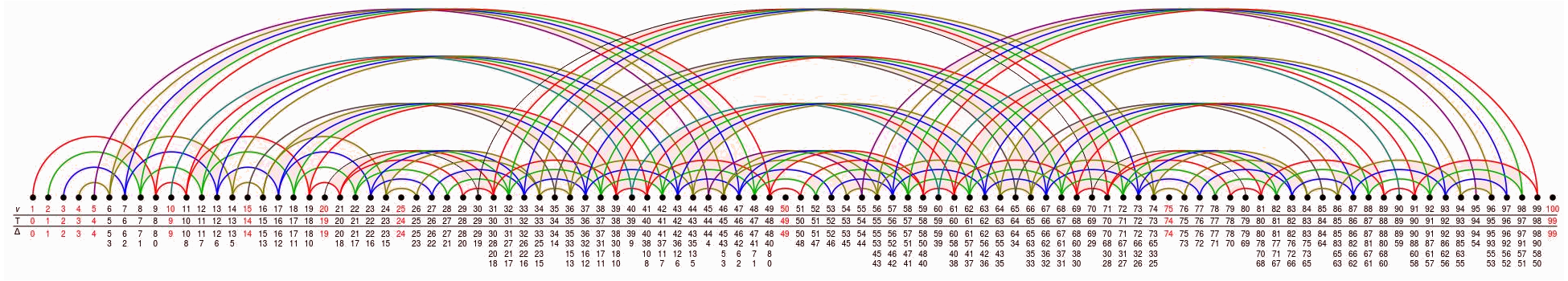## Data

• Title: Quivers for $\mathrm{SL}_{2}$ tilting modules
• Authors: Daniel Tubbenhauer and Paul Wedrich
• Status: Represent. Theory 25 (2021), 440-480. Last update: Mon, 31 May 2021 06:37:56 UTC
• ArXiv version = 0.99 published version
• LaTex Beamer presentation: Slides, Slides2, Slides3

## Abstract

Using diagrammatic methods, we define a quiver algebra depending on a prime $\mathsf{p}$ and show that it is the algebra underlying the category of tilting modules for $\mathrm{SL}_{2}$ in characteristic $\mathsf{p}$. Along the way, we obtain a presentation for morphisms between $\mathsf{p}$-Jones-Wenzl projectors.

## A few extra words

Let $\mathbb{K}$ denote an algebraically closed field and $\mathbf{Tilt}=\mathbf{Tilt}\big(\mathrm{SL}_{2}(\mathbb{K})\big)$ the additive, $\mathbb{K}$-linear category of (left-)tilting modules for the algebraic group $\mathrm{SL}_{2}=\mathrm{SL}_{2}(\mathbb{K})$. This category can be described as the full subcategory of $\mathrm{SL}_{2}$-modules which is monoidally generated by the vector representation $T(1)\cong\mathbb{K}^{2}$, and which is closed under taking finite direct sums and direct summands.
The purpose of this paper is to give a generators and relations presentation of $\mathbf{Tilt}$ by identifying it with the category of projective modules for an explicitly described quiver algebra. For $\mathbb{K}$ of characteristic zero this is trivial as $\mathbf{Tilt}$ is semisimple, and the indecomposable tilting modules are indeed the simple modules. The quantum analog at a complex root of unity is related to the zigzag algebra with vertex set $\mathbb{N}$ and a starting condition.
The focus of this paper is on the case of positive characteristic $\mathsf{p}>0$, for which we represent $\mathbf{Tilt}$ as a quotient $Z=Z_{\mathsf{p}}$ of the path algebra of an infinite, fractal-like quiver, a truncation of which is illustrated for $\mathsf{p}=3$ asThis illustrates the full subquiver containing the first $100$ vertices of the quiver underlying $Z_{3}$.
Note that the algebra $Z$ contains information about the representation theory of $\mathrm{SL}_{2}$, as e.g. about the Weyl factors $\Delta(w_{i}-1)$ in $T(v-1)$. If the $\mathsf{p}$-adic expansion $v=\sum_{i=0}^{j}a_{i}\mathsf{p}^{i}$ has exactly $r+1$ non-zero digits, then there are $2^{r}$ such factors and, correspondingly, $r$ arrows from $v-1$ to certain $w_{i}-1 Note further the uniform behavior of$Z$with respect to$\mathsf{p}$. For exampleis (a cut-off) of the quivers$Z_{2}$,$Z_{5}$and$Z_{7}$, which, zooming out such that the precise labels get invisible, look basically the same as the one for$Z_3$. The basis for our work is the classical fact that the Temperley-Lieb algebra controls the finite-dimensional representation theory of$\mathrm{SL}_{2}$. The second main ingredient is an explicit description of$\mathsf{p}$-Jones--Wenzl projectors, which are characteristic$\mathsf{p}$analogs of the classical Jones-Wenzl projectors. The bulk of this paper is devoted to a careful study of morphisms between$\mathsf{p}$-Jones--Wenzl projectors over$\mathbb{F}_{\mathsf{p}}\$ and the linear relations between them; a result of which we think as being of independent interest.